# 遗传算法

## 1.前言有人说，现代医学阻碍了人类的进化？你怎么看？

## 3．应用领域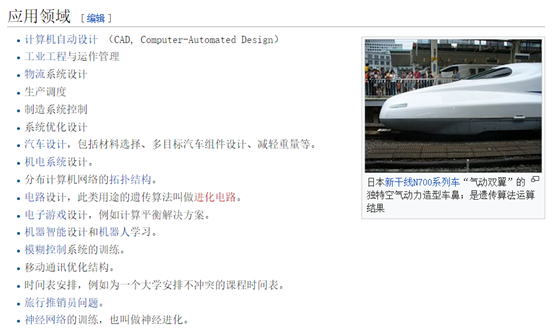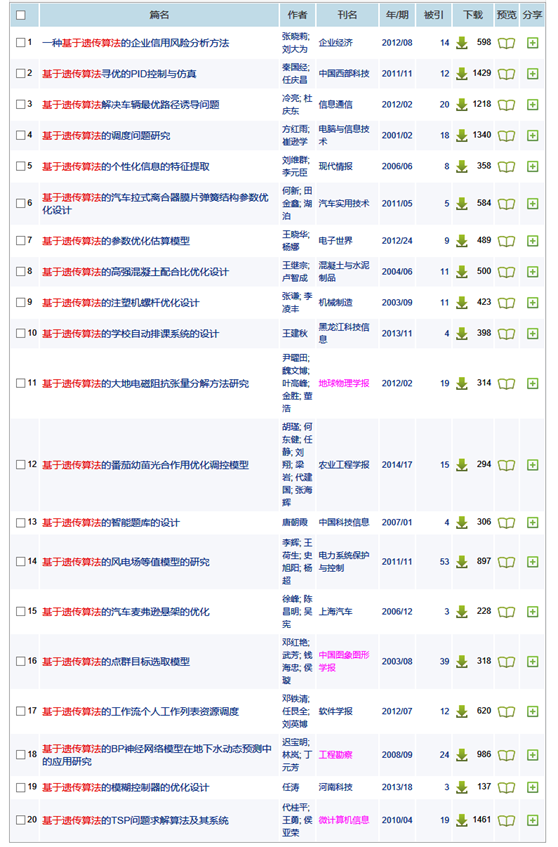## 5．算法详解

### 5.1 对应关系

 生物进化 遗传算法 环境 适应函数 适者生存 适应函数值最大的解被保留的概率最大 个体 问题的一个解 染色体 解的编码 基因 编码的元素 种群 根据适应函数选择的一组解 交叉（交配） 以一定的方式由双亲产生后代的过程 变异 编码的某些分量发生变化的过程

### 5.3流程图描述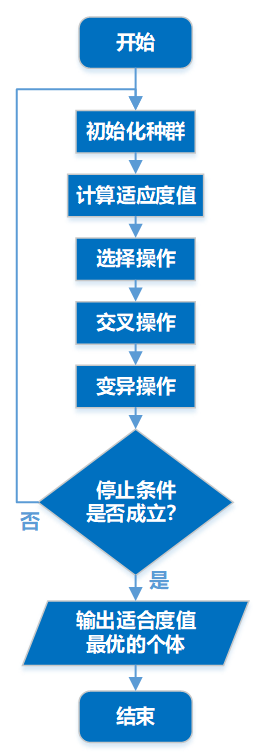### 5.4 伪代码描述

(1) 随机产生初始种群;

(2) 计算种群体中每个个体的适应度值,判断是否满足停

(3) 按由个体适应值所决定的某个规则选择将进入下一代

(4) 按交叉概率Pc进行交叉操作,生产新的个体;

(5) 按变异概率Pm进行变异操作,生产新的个体;

(6) 输出种群中适应度值最优的染色体作为问题的满意解

• Ø 具体讲解

## 6．例题解析

### 6.1 例1：求解多变量多约束非线性规划问题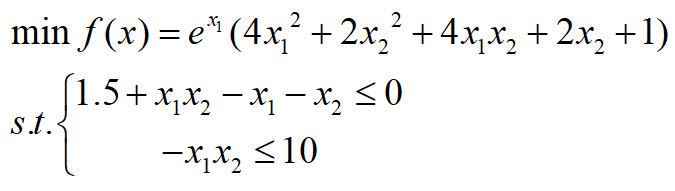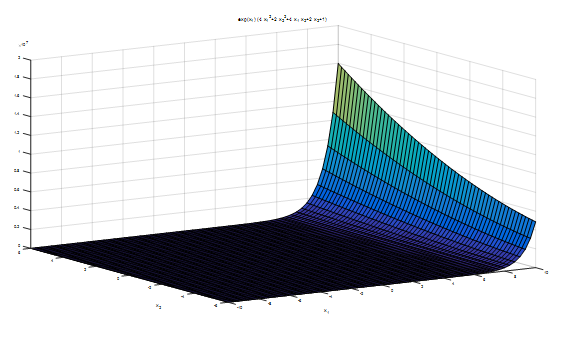这题属于规划类问题，我们可以用LINGO来求解。

(1)  在LINGO中求解：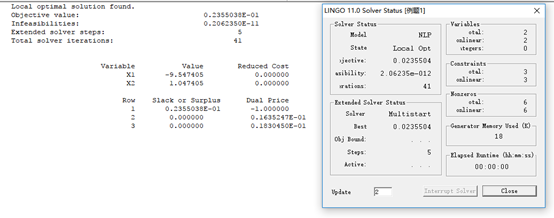(2)  在MATLAB中求解(GA工具箱求解)：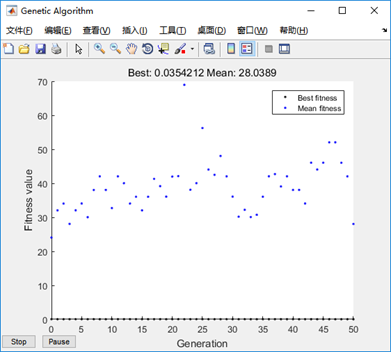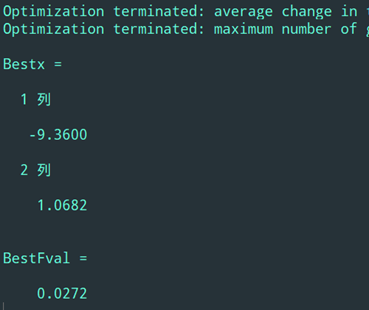### 6.2 例2：求解最值问题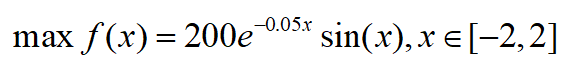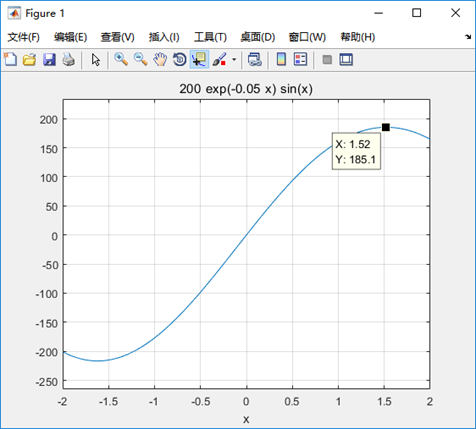(1)   在LINGO中求解：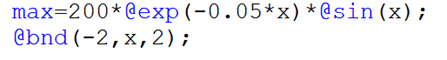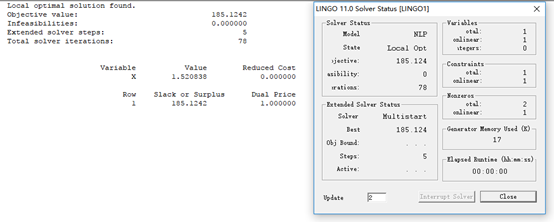(2)  在MATLAB中求解：

%主程序代码,本程序采用遗传算法接力进化，
%将上次进化结束后得到的最终种群作为下次输入的初始种群
%主程序代码,
clc;
close all;
clear all;

% 进化的代数
T=50;
% 初始的种群
options=gaoptimset('Generations',T);
[x,fval,reason,output,final_pop]=ga(@fitnessfun,1,options);

%进行第二次接力进化
options1=gaoptimset('Generations',T,'InitialPopulation',final_pop,'PlotFcns',@gaplotbestf);
[x,fval,reason,output,final_pop]=ga(@fitnessfun,1,options1);

Bestx=x
BestFval=-fval

% 适应度函数
function f = fitnessfun(x)
if(x<-2|x>2)
f=100;
else
f=-200*exp(-0.05*x)*sin(x);
end
end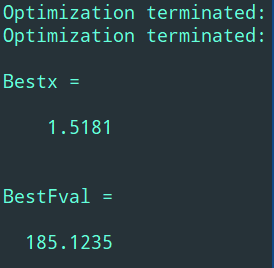%主程序代码,本程序采用遗传算法接力进化，
%将上次进化结束后得到的最终种群作为下次输入的初始种群
clc;
close all;
clear all;

%进化的代数
T=100;
optionsOrigin=gaoptimset('Generations',T);
[x,fval,reason,output,finnal_pop]=ga(@fitnessfun,2,optionsOrigin);

%进行第二次接力进化
options1=gaoptimset('Generations',T,'InitialPopulation',finnal_pop,'PlotFcns',@gaplotbestf);
[x,fval,reason,output,finnal_pop,trace]=ga(@fitnessfun,2,options1);

Bestx=x
BestFval=fval

% 适应度函数
function f = fun(x)
g1=1.5+x(1)*x(2)-x(1)-x(2);
g2=-x(1)*x(2)-10;
if (g1>0|g2>0)
f=100;
else
f=exp(x(1))*(4*x(1)^2+2*x(2)^2+4*x(1)*x(2)+2*x(2)+1);;
end
end

%主程序：
%用遗传算法求解y=200*exp(-0.05*x).*sin(x)在[-2 2]区间上的最大值
clc;
clear all;
close all;

global BitLength
global boundsbegin
global boundsend
bounds=[-2 2];%一维自变量的取值范围
precision=0.0001; %运算精度
boundsbegin=bounds(:,1);
boundsend=bounds(:,2);
%计算如果满足求解精度至少需要多长的染色体
BitLength=ceil(log2((boundsend-boundsbegin)' ./ precision));
popsize=50; %初始种群大小

Generationnmax=100;  %最大代数
pcrossover=0.90; %交配概率
pmutation=0.09; %变异概率
%产生初始种群
population=round(rand(popsize,BitLength));
%计算适应度,返回适应度Fitvalue和累积概率cumsump
[Fitvalue,cumsump]=fitnessfun(population);
Generation=1;
while Generation<Generationnmax+1
for j=1:2:popsize
%选择操作
seln=selection(population,cumsump);
%交叉操作
scro=crossover(population,seln,pcrossover);
scnew(j,:)=scro(1,:);
scnew(j+1,:)=scro(2,:);
%变异操作
smnew(j,:)=mutation(scnew(j,:),pmutation);
smnew(j+1,:)=mutation(scnew(j+1,:),pmutation);
end
population=smnew;  %产生了新的种群
%计算新种群的适应度
[Fitvalue,cumsump]=fitnessfun(population);
%记录当前代最好的适应度和平均适应度
[fmax,nmax]=max(Fitvalue);
fmean=mean(Fitvalue);
ymax(Generation)=fmax;
ymean(Generation)=fmean;
%记录当前代的最佳染色体个体
x=transform2to10(population(nmax,:));
%自变量取值范围是[-2 2],需要把经过遗传运算的最佳染色体整合到[-2 2]区间
xx=boundsbegin+x*(boundsend-boundsbegin)/(power((boundsend),BitLength)-1);
xmax(Generation)=xx;
Generation=Generation+1
end
Generation=Generation-1;
Bestpopulation=xx
Besttargetfunvalue=targetfun(xx)
%绘制经过遗传运算后的适应度曲线。一般地，如果进化过程中种群的平均适应度与最大适
%应度在曲线上有相互趋同的形态，表示算法收敛进行得很顺利，没有出现震荡；在这种前
%提下，最大适应度个体连续若干代都没有发生进化表明种群已经成熟。
figure(1);
hand1=plot(1:Generation,ymax);
set(hand1,'linestyle','-','linewidth',1.8,'marker','*','markersize',6)
hold on;

hand2=plot(1:Generation,ymean);
set(hand2,'color','r','linestyle','-','linewidth',1.8,...
'marker','h','markersize',6)
xlabel('进化代数');ylabel('最大/平均适应度');xlim([1 Generationnmax]);
legend('最大适应度','平均适应度');
box off;hold off;

%子程序：新种群交叉操作,函数名称存储为crossover.m
function scro=crossover(population,seln,pc);
BitLength=size(population,2);
pcc=IfCroIfMut(pc);  %根据交叉概率决定是否进行交叉操作，1则是，0则否
if pcc==1
chb=round(rand*(BitLength-2))+1;  %在[1,BitLength-1]范围内随机产生一个交叉位
scro(1,:)=[population(seln(1),1:chb) population(seln(2),chb+1:BitLength)];
scro(2,:)=[population(seln(2),1:chb) population(seln(1),chb+1:BitLength)];
else
scro(1,:)=population(seln(1),:);
scro(2,:)=population(seln(2),:);
end

%子程序：计算适应度函数, 函数名称存储为fitnessfun
function [Fitvalue,cumsump]=fitnessfun(population);
global BitLength
global boundsbegin
global boundsend
popsize=size(population,1);   %有popsize个个体
for i=1:popsize
x=transform2to10(population(i,:));  %将二进制转换为十进制
%转化为[-2,2]区间的实数
xx=boundsbegin+x*(boundsend-boundsbegin)/(power((boundsend),BitLength)-1);
Fitvalue(i)=targetfun(xx);  %计算函数值，即适应度
end
%给适应度函数加上一个大小合理的数以便保证种群适应值为正数
Fitvalue=Fitvalue'+230;
%计算选择概率
fsum=sum(Fitvalue);
Pperpopulation=Fitvalue/fsum;
%计算累积概率
cumsump(1)=Pperpopulation(1);
for i=2:popsize
cumsump(i)=cumsump(i-1)+Pperpopulation(i);
end
cumsump=cumsump';

%子程序：新种群变异操作，函数名称存储为mutation.m
function snnew=mutation(snew,pmutation);
BitLength=size(snew,2);
snnew=snew;
pmm=IfCroIfMut(pmutation);  %根据变异概率决定是否进行变异操作，1则是，0则否
if pmm==1
chb=round(rand*(BitLength-1))+1;  %在[1,BitLength]范围内随机产生一个变异位
snnew(chb)=abs(snew(chb)-1);
end

%子程序：判断遗传运算是否需要进行交叉或变异, 函数名称存储为IfCroIfMut.m
function pcc=IfCroIfMut(mutORcro);
test(1:100)=0;
l=round(100*mutORcro);
test(1:l)=1;
n=round(rand*99)+1;
pcc=test(n);

%子程序：新种群选择操作, 函数名称存储为selection.m
function seln=selection(population,cumsump);
%从种群中选择两个个体
for i=1:2
r=rand;  %产生一个随机数
prand=cumsump-r;
j=1;
while prand(j)<0
j=j+1;
end
seln(i)=j; %选中个体的序号
end

%子程序：将2进制数转换为10进制数,函数名称存储为transform2to10.m
function x=transform2to10(Population);
BitLength=size(Population,2);
x=Population(BitLength);
for i=1:BitLength-1
x=x+Population(BitLength-i)*power(2,i);
end

%子程序：对于优化最大值或极大值函数问题，目标函数可以作为适应度函数
%函数名称存储为targetfun.m
function y=targetfun(x); %目标函数
y=200*exp(-0.05*x).*sin(x);©️2020 CSDN 皮肤主题: 大白 设计师: CSDN官方博客点击重新获取扫码支付1.余额是钱包充值的虚拟货币，按照1:1的比例进行支付金额的抵扣。
2.余额无法直接购买下载，可以购买VIP、C币套餐、付费专栏及课程。余额充值